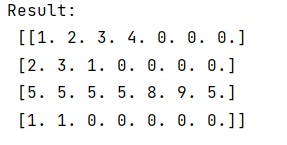# How to fix array with rows of different lengths by filling the empty elements with zeros?

Learn, how can we fix array with rows of different lengths by filling the empty elements with zeros?
Submitted by Pranit Sharma, on March 07, 2023

## Initializing empty array of given length

Suppose that we are given an array where each row has a different length and we need to fill the empty elements with zero to make the length of all rows equal.

To fix array with rows of different lengths by filling the empty elements with zeros, we can define a function where we will first extract the length of all the rows and then we will create a mask of length wherever the filling of zeroes is necessary.

We will then set up an output array and put elements from data into masked positions and call this function to fill the rows with zeros.

Let us understand with the help of an example,

## Python code to fix array with rows of different lengths by filling the empty elements with zeros

```# Import numpy
import numpy as np

# Defining a function
def fun(a):
leng = np.array([len(i) for i in a])
return res

# Filling required rows with 0's
res = fun([[1, 2, 3, 4],[2, 3, 1],[5, 5, 5, 5, 8 ,9 ,5],[1, 1]])

# Display result
print("Result:\n",res,"\n")
```

Output:Languages: » C » C++ » C++ STL » Java » Data Structure » C#.Net » Android » Kotlin » SQL
Web Technologies: » PHP » Python » JavaScript » CSS » Ajax » Node.js » Web programming/HTML
Solved programs: » C » C++ » DS » Java » C#
Aptitude que. & ans.: » C » C++ » Java » DBMS
Interview que. & ans.: » C » Embedded C » Java » SEO » HR
CS Subjects: » CS Basics » O.S. » Networks » DBMS » Embedded Systems » Cloud Computing
» Machine learning » CS Organizations » Linux » DOS
More: » Articles » Puzzles » News/Updates